# Number to Words Converter in Excel - Indian Rupees

To convert a Number in Words in MS Excel you have to use Macro, It's very simple and with few steps given below, you can create a macro function in MS Excel for converting Numbers into words in rupees. Once you have to follow given simple steps and you will get a Permanent Formula/Function for Number to word's converter in Indian Rupees Format. It will be use as function so you can convert your any column data by just drag and drop the formula.

This function/macro is useful for Indian Number Format like - Lakh, Crore, Arab, Kharb. For International (Million-Billion) format click here.

Steps for Number to Word Converter in MS Excel - Indian (Lakh, Crore Format)

Step 1 - Start MS Excel

Step 2 - Just Type Sample Number's in one column (see below image) to convert into Words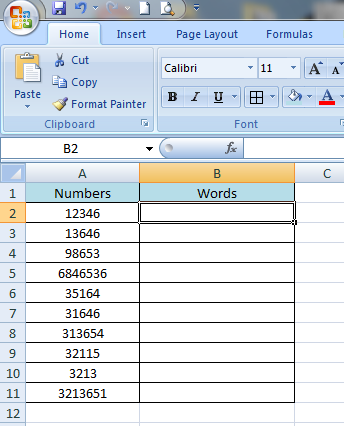Step 3 - Press ALT+F11 to start the Visual Basic Editor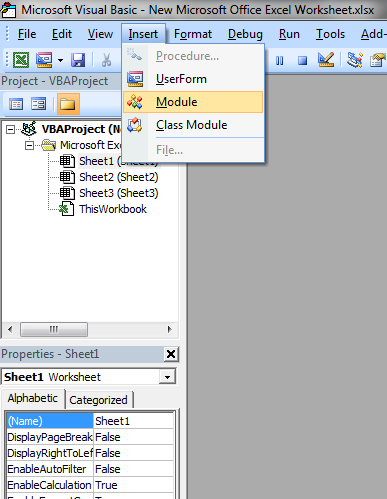Step 4 - You will see a Window is Open, Now copy-paste following code in this window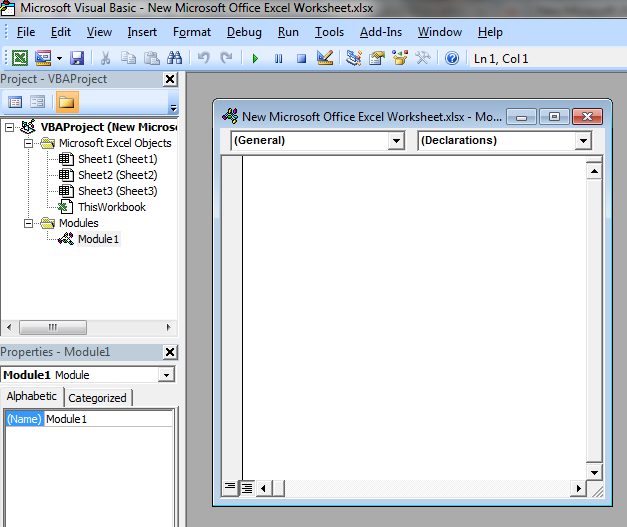``Option Explicit'Main Function`Function ConvertCurrencyToEnglish(ByVal MyNumber) Dim Temp Dim Rupees, Paise Dim DecimalPlace, Count  ReDim Place(9) As String Place(2) = " Thousand " Place(3) = " lakh " Place(4) = " Crore "   ' Convert MyNumber to a string, trimming extra spaces. MyNumber = Trim(Str(MyNumber))  ' Find decimal place. DecimalPlace = InStr(MyNumber, ".")  ' If we find decimal place... If DecimalPlace > 0 Then ' Convert Paise Temp = Left(Mid(MyNumber, DecimalPlace + 1) & "00", 2) ' Hi! Note the above line Mid function it gives right portion ' after the decimal point 'if only . and no numbers such as 789. accures, mid returns nothing ' to avoid error we added 00 ' Left function gives only left portion of the string with specified places here 2   Paise = ConvertTens(Temp)   ' Strip off paise from remainder to convert. MyNumber = Trim(Left(MyNumber, DecimalPlace - 1)) End If  Count = 1 If MyNumber <> "" Then  ' Convert last 3 digits of MyNumber to Indian Rupees. Temp = ConvertHundreds(Right(MyNumber, 3))  If Temp <> "" Then Rupees = Temp & Place(Count) & Rupees  If Len(MyNumber) > 3 Then ' Remove last 3 converted digits from MyNumber. MyNumber = Left(MyNumber, Len(MyNumber) - 3) Else MyNumber = "" End If  End If  ' convert last two digits to of mynumber Count = 2  Do While MyNumber <> "" Temp = ConvertTens(Right("0" & MyNumber, 2))  If Temp <> "" Then Rupees = Temp & Place(Count) & Rupees If Len(MyNumber) > 2 Then ' Remove last 2 converted digits from MyNumber. MyNumber = Left(MyNumber, Len(MyNumber) - 2)  Else MyNumber = "" End If Count = Count + 1  Loop  ' Clean up rupees. Select Case Rupees Case "" Rupees = "" Case "One" Rupees = "Rupee One" Case Else Rupees = "Rupees " & Rupees End Select  ' Clean up paise. Select Case Paise Case "" Paise = "" Case "One" Paise = "One Paise" Case Else Paise = Paise & " Paise" End Select  If Rupees = "" Then ConvertCurrencyToEnglish = Paise & " Only" ElseIf Paise = "" Then ConvertCurrencyToEnglish = Rupees & " Only" Else ConvertCurrencyToEnglish = Rupees & " and " & Paise & " Only" End If End Function Private Function ConvertDigit(ByVal MyDigit) Select Case Val(MyDigit) Case 1: ConvertDigit = "One" Case 2: ConvertDigit = "Two" Case 3: ConvertDigit = "Three" Case 4: ConvertDigit = "Four" Case 5: ConvertDigit = "Five" Case 6: ConvertDigit = "Six" Case 7: ConvertDigit = "Seven" Case 8: ConvertDigit = "Eight" Case 9: ConvertDigit = "Nine" Case Else: ConvertDigit = "" End Select End Function Private Function ConvertHundreds(ByVal MyNumber) Dim Result As String  ' Exit if there is nothing to convert. If Val(MyNumber) = 0 Then Exit Function  ' Append leading zeros to number. MyNumber = Right("000" & MyNumber, 3)  ' Do we have a hundreds place digit to convert? If Left(MyNumber, 1) <> "0" Then Result = ConvertDigit(Left(MyNumber, 1)) & " Hundred " End If  ' Do we have a tens place digit to convert? If Mid(MyNumber, 2, 1) <> "0" Then Result = Result & ConvertTens(Mid(MyNumber, 2)) Else ' If not, then convert the ones place digit. Result = Result & ConvertDigit(Mid(MyNumber, 3)) End If  ConvertHundreds = Trim(Result)End Function Private Function ConvertTens(ByVal MyTens) Dim Result As String  ' Is value between 10 and 19? If Val(Left(MyTens, 1)) = 1 Then Select Case Val(MyTens) Case 10: Result = "Ten" Case 11: Result = "Eleven" Case 12: Result = "Twelve" Case 13: Result = "Thirteen" Case 14: Result = "Fourteen" Case 15: Result = "Fifteen" Case 16: Result = "Sixteen" Case 17: Result = "Seventeen" Case 18: Result = "Eighteen" Case 19: Result = "Nineteen" Case Else End Select Else ' .. otherwise it's between 20 and 99. Select Case Val(Left(MyTens, 1)) Case 2: Result = "Twenty " Case 3: Result = "Thirty " Case 4: Result = "Forty " Case 5: Result = "Fifty " Case 6: Result = "Sixty " Case 7: Result = "Seventy " Case 8: Result = "Eighty " Case 9: Result = "Ninety " Case Else End Select  ' Convert ones place digit. Result = Result & ConvertDigit(Right(MyTens, 1)) End If  ConvertTens = ResultEnd Function`

Step 5 - Save this by clicking on save in window with following format, Excel macro Enabled Workbook.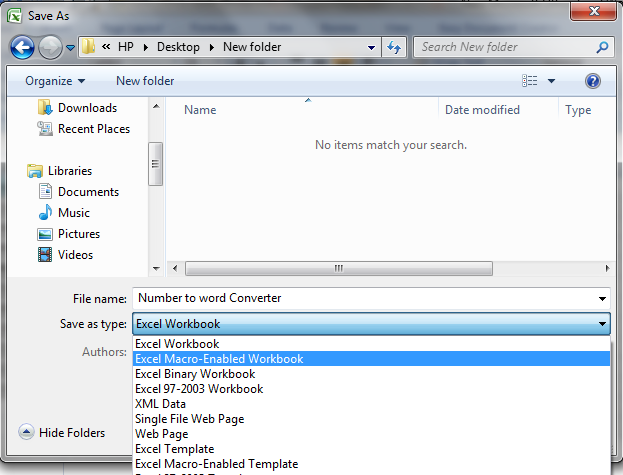Step 6 - Now come again on your original excel sheet on which you have to convert Number in Words.
Step 7 - Just Click on Formulas then -> Insert Function Option, A window will open.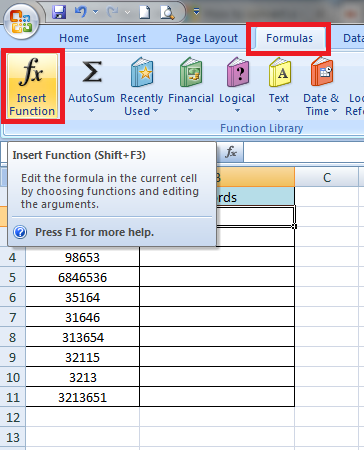Step 8 - In Drop Down Box Select "User Defined".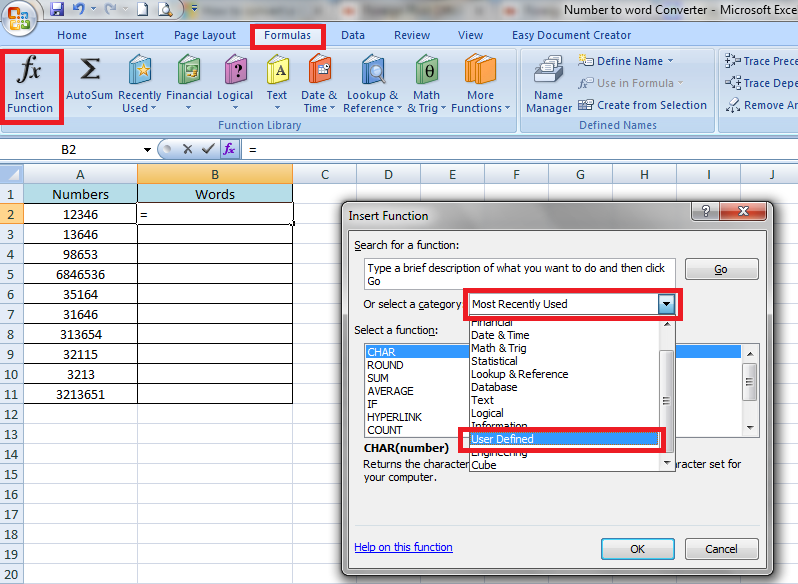Step 9 - Now Select "ConvertCurrencyToEnglish" and Click on OK.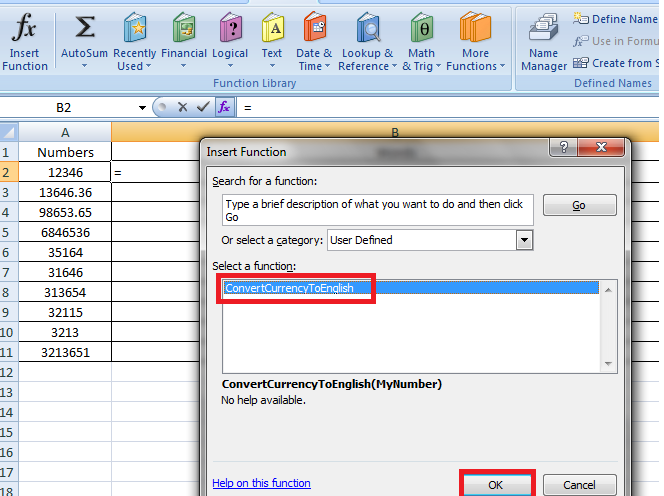Step  10 - Now a New Window will Show Click on button shown as below and Select Number Column A1. Click on OK. You will see Number is converted in Words.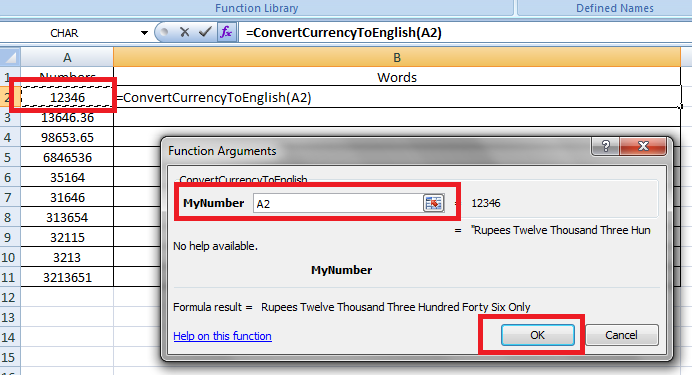Step 11 - Now your number will converted in words. You can drag and drop to apply this converter formula on all of the numbers.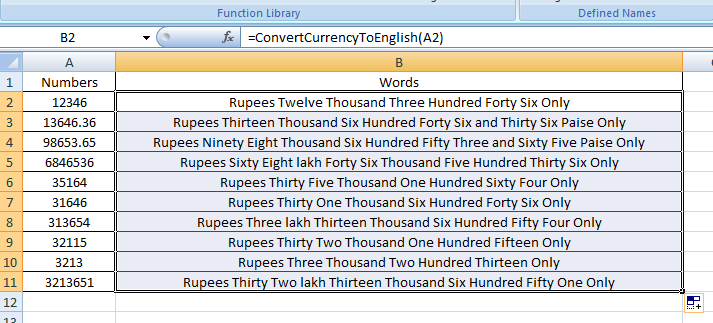Step 12 - Now you can use this Macro Excel sheet to use Formula you have Made any time any where in Excel Sheet.